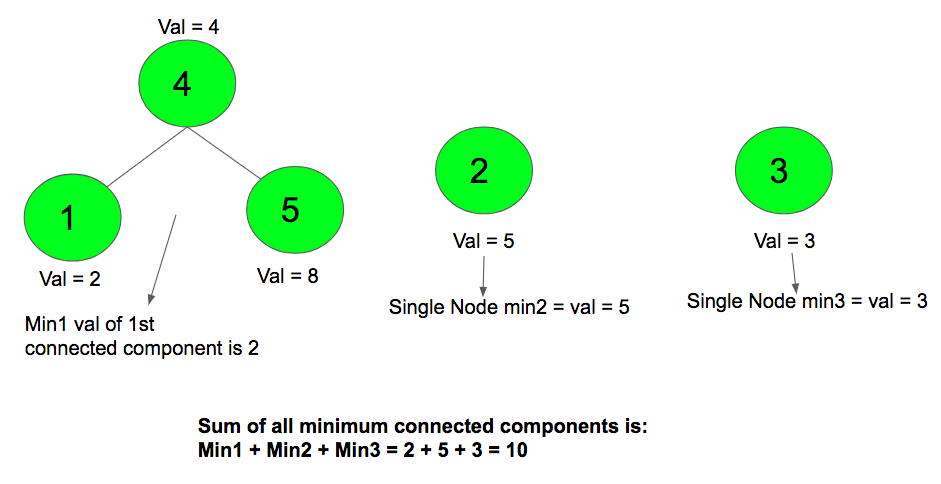# Sum of the minimum elements in all connected components of an undirected graph

Given an array A of N numbers where Ai represent the value of the (i+1)th node. Also given are M pair of edges where u and v represent the nodes that are connected by an edge. The task is to find the sum of the minimum element in all the connected components of the given undirected graph. If a node has no connectivity to any other node, count it as a component with one node.

Examples:

Input: a[] = {1, 6, 2, 7, 3, 8, 4, 9, 5, 10} m = 5
1 2
3 4
5 6
7 8
9 10

Output: 15
Connected components are: 1–2, 3–4, 5–6, 7–8 and 9–10
Sum of Minimum of all them : 1 + 2 + 3 + 4 + 5 = 15

Input: a[] = {2, 5, 3, 4, 8} m = 2
1 4
4 5
Output: 10## Recommended: Please try your approach on {IDE} first, before moving on to the solution.

Approach: Finding connected components for an undirected graph is an easier task. Doing either a BFS or DFS starting from every unvisited vertex will give us our connected components. Create a visited[] array which has initially all nodes marked as False. Iterate all the nodes, if the node is not visited, call DFS() function so that all the nodes connected directly or indirectly to the node are marked as visited. While visiting all the directly or indirectly connected nodes, store the minimum value of all nodes. Create a variable sum which stores the summation of the minimum of all these connected components. Once all the nodes are visited, sum will have the answer to the problem.

Below is the implementation of the above approach:

 `// C++ program to find the sum ` `// of the minimum elements in all ` `// connected components of an undirected graph ` `#include ` `using` `namespace` `std; ` `const` `int` `N = 10000; ` `vector<``int``> graph[N]; ` ` `  `// Initially all nodes ` `// marked as unvisited ` `bool` `visited[N]; ` ` `  `// DFS function that visits all ` `// connected nodes from a given node ` `void` `dfs(``int` `node, ``int` `a[], ``int` `mini) ` `{ ` `    ``// Stores the minimum ` `    ``mini = min(mini, a[node]); ` ` `  `    ``// Marks node as visited ` `    ``visited[node] = ``true``; ` ` `  `    ``// Traversed in all the connected nodes ` `    ``for` `(``int` `i : graph[node]) { ` `        ``if` `(!visited[i]) ` `            ``dfs(i, a, mini); ` `    ``} ` `} ` ` `  `// Function to add the edges ` `void` `addedge(``int` `u, ``int` `v) ` `{ ` `    ``graph[u - 1].push_back(v - 1); ` `    ``graph[v - 1].push_back(u - 1); ` `} ` ` `  `// Function that returns the sum of all minimums ` `// of connected componenets of graph ` `int` `minimumSumConnectedComponents(``int` `a[], ``int` `n) ` `{ ` `    ``// Initially sum is 0 ` `    ``int` `sum = 0; ` ` `  `    ``// Traverse for all nodes ` `    ``for` `(``int` `i = 0; i < n; i++) { ` `        ``if` `(!visited[i]) { ` `            ``int` `mini = a[i]; ` `            ``dfs(i, a, mini); ` `            ``sum += mini; ` `        ``} ` `    ``} ` `     `  `    ``// Returns the answer ` `    ``return` `sum; ` `} ` ` `  `// Driver Code ` `int` `main() ` `{ ` `    ``int` `a[] = {1, 6, 2, 7, 3, 8, 4, 9, 5, 10}; ` `     `  `    ``// Add edges ` `    ``addedge(1, 2); ` `    ``addedge(3, 4); ` `    ``addedge(5, 6); ` `    ``addedge(7, 8); ` `    ``addedge(9, 10); ` `     `  `    ``int` `n = ``sizeof``(a) / ``sizeof``(a); ` ` `  `    ``// Calling Function ` `    ``cout << minimumSumConnectedComponents(a, n); ` `    ``return` `0; ` `} `

Output:

```15
```

Don’t stop now and take your learning to the next level. Learn all the important concepts of Data Structures and Algorithms with the help of the most trusted course: DSA Self Paced. Become industry ready at a student-friendly price.

My Personal Notes arrow_drop_upCheck out this Author's contributed articles.

If you like GeeksforGeeks and would like to contribute, you can also write an article using contribute.geeksforgeeks.org or mail your article to contribute@geeksforgeeks.org. See your article appearing on the GeeksforGeeks main page and help other Geeks.

Please Improve this article if you find anything incorrect by clicking on the "Improve Article" button below.